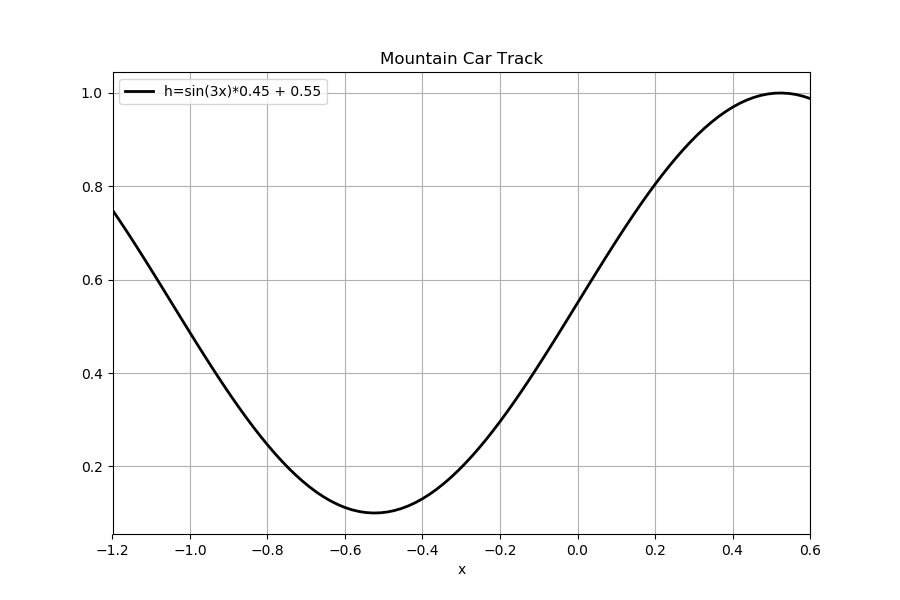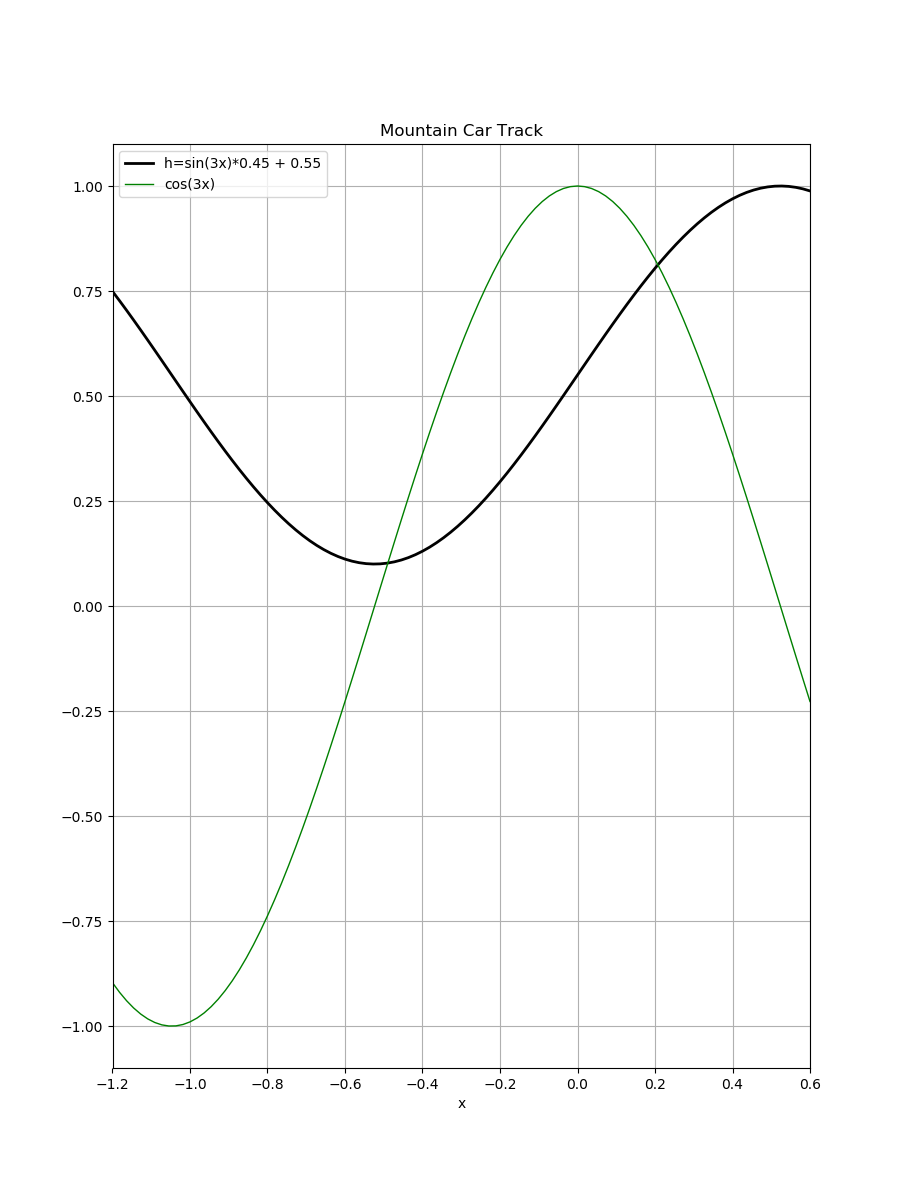# # Mountain Car的环境分析

$h = 0.45\sin(3x)+0.55$

import numpy as np
import matplotlib.pyplot as plt
import os

save_path = './img/'
if not os.path.exists(save_path):
os.mkdir(save_path)

SIZE = 1.5
plt.figure(figsize=(SIZE*6,SIZE*4))
plt.xlabel('x')
plt.title('Mountain Car Track')
plt.xlim(-1.2,0.6)
x = np.linspace(-1.2,0.6,100)
plt.plot(x, np.sin(3*x)*0.45 + 0.55, 'k-',lw=2,label='h=sin(3x)*0.45 + 0.55')
plt.legend(loc='upper left')
plt.grid()
plt.savefig(os.path.join(save_path,'MountainCarTrack'))
plt.show()

1
2
3
4
5
6
7
8
9
10
11
12
13
14
15
16
17
18
19• 轨道的表达式$h = 0.45\sin(3x)+0.55$;
• 车的质量$m=1$;
• 车受到的重力$\text{gravity} = 0.0025$;
• 位置$x$的范围$[-1.2, 0.5]$;
• 速度$v$的范围$[-0.07,0.07]$;
• 智能体在任意时刻$t$可采取的动作$A_t \in \{0,1,2\}$;
• 智能体的驱动力为$f = (A_t - 1)*0.001$;
• 智能体的状态$(x,v)$
• 终点位置$x=0.5$

$v_{t+1} \doteq \text{bound}[v_t + 0.001(A_t-1) - 0.0025\cos(3x_t)]$

$x_{t+1} \doteq \text{bound}[x_{t} + v_{t+1}]$

$v$的递推关系来看, 我们可以认为速度$v$的方向是沿着轨道的; 接下来我们认为小车的驱动力$f$的方向也是沿着轨道的。$x_{t+1} \doteq \text{bound}[x_{t} + v_{t+1}]$